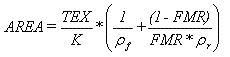# Tow area calculationArea Area of tow bundle

Tex Total Tex of fibres in bundle. e.g. 2 fibres of 2400 Tex = 4800.

ρf      Resin Density.

ρr     Fibre Density.

K Area calculation constant.

FMR Mass fraction of fibres in composite.

For the Area calculated to be in correct units, the formula is required to produce an area in the same units as the mandrel was initially generated in. Thus the units of TEX, Fraction Mass Ratio, etc., need to be consistent.

Example :Densities Grams Per Cubic Centimetre.

TEX Grams Per Kilometre.

K=1000

Updated: June 2020